Test: Digital Transmission

# Test: Digital Transmission - Electronics and Communication Engineering (ECE)

Test Description

## 20 Questions MCQ Test GATE ECE (Electronics) 2023 Mock Test Series - Test: Digital Transmission

Test: Digital Transmission for Electronics and Communication Engineering (ECE) 2023 is part of GATE ECE (Electronics) 2023 Mock Test Series preparation. The Test: Digital Transmission questions and answers have been prepared according to the Electronics and Communication Engineering (ECE) exam syllabus.The Test: Digital Transmission MCQs are made for Electronics and Communication Engineering (ECE) 2023 Exam. Find important definitions, questions, notes, meanings, examples, exercises, MCQs and online tests for Test: Digital Transmission below.
Solutions of Test: Digital Transmission questions in English are available as part of our GATE ECE (Electronics) 2023 Mock Test Series for Electronics and Communication Engineering (ECE) & Test: Digital Transmission solutions in Hindi for GATE ECE (Electronics) 2023 Mock Test Series course. Download more important topics, notes, lectures and mock test series for Electronics and Communication Engineering (ECE) Exam by signing up for free. Attempt Test: Digital Transmission | 20 questions in 60 minutes | Mock test for Electronics and Communication Engineering (ECE) preparation | Free important questions MCQ to study GATE ECE (Electronics) 2023 Mock Test Series for Electronics and Communication Engineering (ECE) Exam | Download free PDF with solutions
 1 Crore+ students have signed up on EduRev. Have you?
Test: Digital Transmission - Question 1

### A linear delta modulator is designed to operate on speech signals limited to 3.4 kHz. The sampling rate is 10 time the Nyquist rate of the speech signal. The step size δ  is 100 mV. The modulator is tested with a this test signal required to avoid slope overload is

Detailed Solution for Test: Digital Transmission - Question 1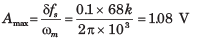Test: Digital Transmission - Question 2

### Consider a linear DM system designed to accommodate analog message signals limited to bandwidth of 3.5 kHz. A sinusoidal test signals of amplitude Amax = 1 V and frequency fm = 800 Hz is applied to system. The sampling rate of the system is 64 kHz. Que: The minimum value of the step size to avoid overload is

Detailed Solution for Test: Digital Transmission - Question 2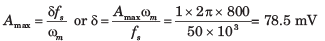Test: Digital Transmission - Question 3

### Consider a linear DM system designed to accommodate analog message signals limited to bandwidth of 3.5 kHz. A sinusoidal test signals of amplitude Amax = 1 V and frequency fm = 800 Hz is applied to system. The sampling rate of the system is 64 kHz. Que: The granular-noise power would be

Detailed Solution for Test: Digital Transmission - Question 3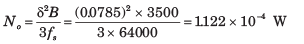Test: Digital Transmission - Question 4

The SNR will be

Detailed Solution for Test: Digital Transmission - Question 4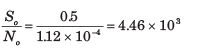Test: Digital Transmission - Question 5

The output signal-to-quantization-noise ratio of a 10-bit PCM was found to be 30 dB. The desired SNR is 42 dB. It can be increased by increasing the number of quantization level.In this way the fractional increase in the transmission bandwidth would be (assume log210 = 0.3)

Detailed Solution for Test: Digital Transmission - Question 5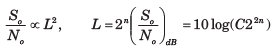= log C + 20 nlog2  =   α + 6 ndB. This equation shows that increasing n by one bits increase the by 6 dB.
Hence an increase in the SNR by 12 dB can be accomplished by increasing 9is form 10 to 12, the transmission bandwidth would be increased by 20%

Test: Digital Transmission - Question 6

A signal has a bandwidth of 1 MHz. It is sampled at a rate 50% higher than the Nyquist rate and  quantized into 256 level using a  -low quantizer with μ = 225.

Que: The signal-to-quantization-noise ratio is

Detailed Solution for Test: Digital Transmission - Question 6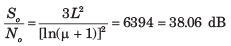Test: Digital Transmission - Question 7

A signal has a bandwidth of 1 MHz. It is sampled at a rate 50% higher than the Nyquist rate and  quantized into 256 level using a  -low quantizer with μ = 225.

Que: It was found that a sampling rate 20% above therate would be adequate. So the maximum SNR, thatcan be realized without increasing the transmission bandwidth, would be

Detailed Solution for Test: Digital Transmission - Question 7

Nyquist Rate = 2 MHz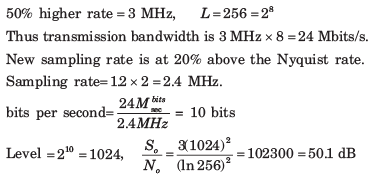Test: Digital Transmission - Question 8

For a PCM signal the compression parameter μ = 100 and the minimum signal to quantization-noiseratio is 50 dB. The number of bits per sample would be.

Detailed Solution for Test: Digital Transmission - Question 8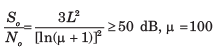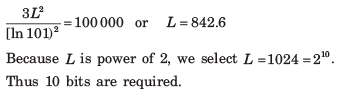Test: Digital Transmission - Question 9

A sinusoid massage signal m(t) is transmitted by binary PCM without compression. If the signalto-quantization-noise ratio is required to be at least 48dB, the minimum number of bits per sample will be

Detailed Solution for Test: Digital Transmission - Question 9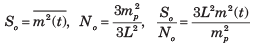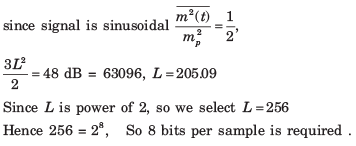Test: Digital Transmission - Question 10

A speech signal has a total duration of 20 sec. It is sampled at the rate of 8 kHz and then PCM encoded. The signal-to-quantization noise ratio is required to be 40dB. The minimum storage capacity needed to accommodate this signal is

Detailed Solution for Test: Digital Transmission - Question 10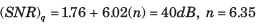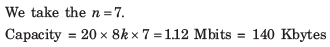Test: Digital Transmission - Question 11

The input to a linear delta modulator having fstep-size Δ = 0.628 is a sine wave with frequency fm and peak amplitude Em. If the sampling frequency fs = 40 kHz, the combination of the sinc-wave frequency and the peak amplitude, where slope overload will take place is

Detailed Solution for Test: Digital Transmission - Question 11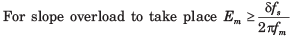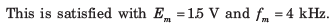Test: Digital Transmission - Question 12

A sinusoidal signal with peak-to-peak amplitude of 1.536 V is quantized into 128 levels using a mid-rise uniform quantizer. The quantization-noise power is

Detailed Solution for Test: Digital Transmission - Question 12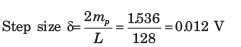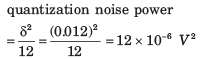Test: Digital Transmission - Question 13

signal is sampled at 8 kHz and is quantized using 8 bit uniform quantizer. Assuming SNRq for a sinusoidal signal, the correct statement for PCM signal with a bit rate of R is

Detailed Solution for Test: Digital Transmission - Question 13

Bit Rate = 8k x 8 = 64 kbps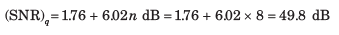Test: Digital Transmission - Question 14

A 1.0 kHz signal is flat-top sampled at the rate of 180 samples sec and the samples are applied to an idealrectangular LPF with cat-off frequency of 1100 Hz, then the output of the filter contains

Detailed Solution for Test: Digital Transmission - Question 14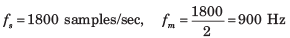Since the sampling rate is 1800 samples/sec the highest frequency that can be recovered is 900 Hz.

Test: Digital Transmission - Question 15

The Nyquist sampling interval, for the signal sinc(700t) + sinc(500t) is

Detailed Solution for Test: Digital Transmission - Question 15

x(t) = sinc 700t + sinc 500t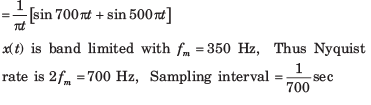Test: Digital Transmission - Question 16

A signal x(t) = 100 cos(24π x 103)t is ideally sampled with a sampling period of 50 sec and then passed through an ideal lowpass filter with cutoff frequency of 15 KHz. Which of the following frequencies is/are present at the filter output

Detailed Solution for Test: Digital Transmission - Question 16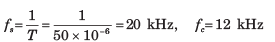The frequency passed through LPF are fc ,fs - fm or 12 kHz, 8 kHz

Test: Digital Transmission - Question 17

In a PCM system, if the code word length is increased form 6 to 8 bits, the signal to quantization noise ratio improves by the factor.

Detailed Solution for Test: Digital Transmission - Question 17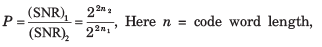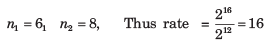Test: Digital Transmission - Question 18

Four signals g1(t) , g3(t), g2(t) and g4(t) are to be multiplexed and transmitted. g1(t)  and g4(t) have a bandwidth of 4 kHz, and the remaining two signals have bandwidth of 8 kHz,. Each sample requires 8 bit for encoding. What is the minimum transmission bit rate of the system.

Detailed Solution for Test: Digital Transmission - Question 18

signals g1(t) , g3(t), g2(t) and g4(t) will have 8 k, 8 k, 16 k and 16 k sample/sec at Nyquist rate. Thus 48000 sample/sec bit rate 48000 x  8 =384 kbps

Test: Digital Transmission - Question 19

Three analog signals, having bandwidths 1200 Hz, 600 Hz and 600 Hz, are sampled at their respective Nyquist rates, encoded with 12 bit words, and time division multiplexed. The bit rate for the multiplexed signal is

Detailed Solution for Test: Digital Transmission - Question 19

Analog signals, having bandwidth 1200 Hz, 600 Hz and 600 Hz have 2400, 1200 samples/sec at Nyquist rate. Hence 48000 sample/sec

bit rate  = 48000 sample/sec x 12 = 57.6 kbps

Test: Digital Transmission - Question 20

The minimum sampling frequency (in samples/sec) required to reconstruct the following signal form its samples without distortion would be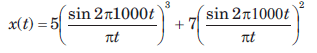Detailed Solution for Test: Digital Transmission - Question 20Maximum frequency component = 3 x 1000 = 3 kHz Sampling rate = 2 fm = 6kHz

## GATE ECE (Electronics) 2023 Mock Test Series

21 docs|263 tests
Information about Test: Digital Transmission Page
In this test you can find the Exam questions for Test: Digital Transmission solved & explained in the simplest way possible. Besides giving Questions and answers for Test: Digital Transmission , EduRev gives you an ample number of Online tests for practice

## GATE ECE (Electronics) 2023 Mock Test Series

21 docs|263 tests(Scan QR code)